SYLLABUS  Previous: 2.1 Explicit 2 levels  Up: 2 FINITE DIFFERENCES  Next: 2.3 Lax-Wendroff

## 2.2 Explicit 3 levels

Slide : [ 3 levels Scheme - Code - Run || VIDEO login]

A more accurate scheme in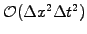is obtained from centered differences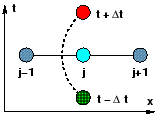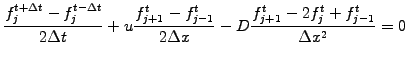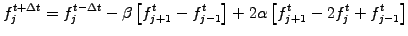(2.2.0#eq.5)

The scheme involves three time levels (fm[j], f[j], fp[j]) and has been implemented in JBONE as

      for (int j=1; j<n; j++) {
fp[j]=fm[j] -beta*(f[j+1]-f[j-1]) +2*alpha*(f[j+1]-2.*f[j]+f[j-1]);}
fp=fm   -beta*(f[ 1 ]-f[ n ]) +2*alpha*(f[ 1 ]-2.*f+f[ n ]);
fp[n]=fm[n]   -beta*(f[ 0 ]-f[n-1]) +2*alpha*(f[ 0 ]-2.*f[n]+f[n-1]);

A special starting procedure is required to calculate an approximation at a time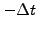anterior to the initial condition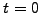. This can be implemented by taking one step backwards in time with the explicit 2 levels scheme (2.1#eq.1). The example below shows the result obtained using the same parameters for the advection equation as in the previous section.

Despite the mathematically higher accuracy inand the excellent conservation of the lowest order moment, the initial box function is strongly distorted by the phase errors created from numerical dispersion, when short wavelengths propagate at a different speed from longuer wavelengths due to inaccuracies in the finite difference approximations.

 Numerical experiments: explicit 3-levels Switch to Cosine and check how the scheme performs for the advection of harmonic functions with ICWavelength=4 mesh points per wavelength. Measure the propagation speed and compare with the physical Velocity that is given as an input parameter. What happens with ICWavelength=2 mesh point per wavelength? Switch to a Gaussian initial condition and observe how the pulse propagates accross the periodic domain. Explain how the initial pulse gets distorted. Switch to Explicit 2-level and compare the numerical dispersion / damping in 2 and 3 levels schemes. Back to Explicit 3-level, change the sign of Velocity=-1 and verify that a symmetric scheme indeed works for both directions. Add a small physical Diffusion=0.1 and verify that the same spatial difference used in (2.1#eq.1) is here in fact always unstable (exercise 2.02).

Because the numerical dispersion slows down short wavelengths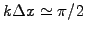, the solution can become locally negative even if it was strictly positive at the beginning. This is of course not physical and can be a problem if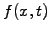is a density... Low numerical damping, however, makes the 3 levels scheme attractive when a simple solution is sought only for advection. The choice between a 2 or 3 level scheme ultimately depends on the specific application and always implies a sound monitoring of the numerical parameters at run time.

SYLLABUS  Previous: 2.1 Explicit 2 levels  Up: 2 FINITE DIFFERENCES  Next: 2.3 Lax-Wendroff